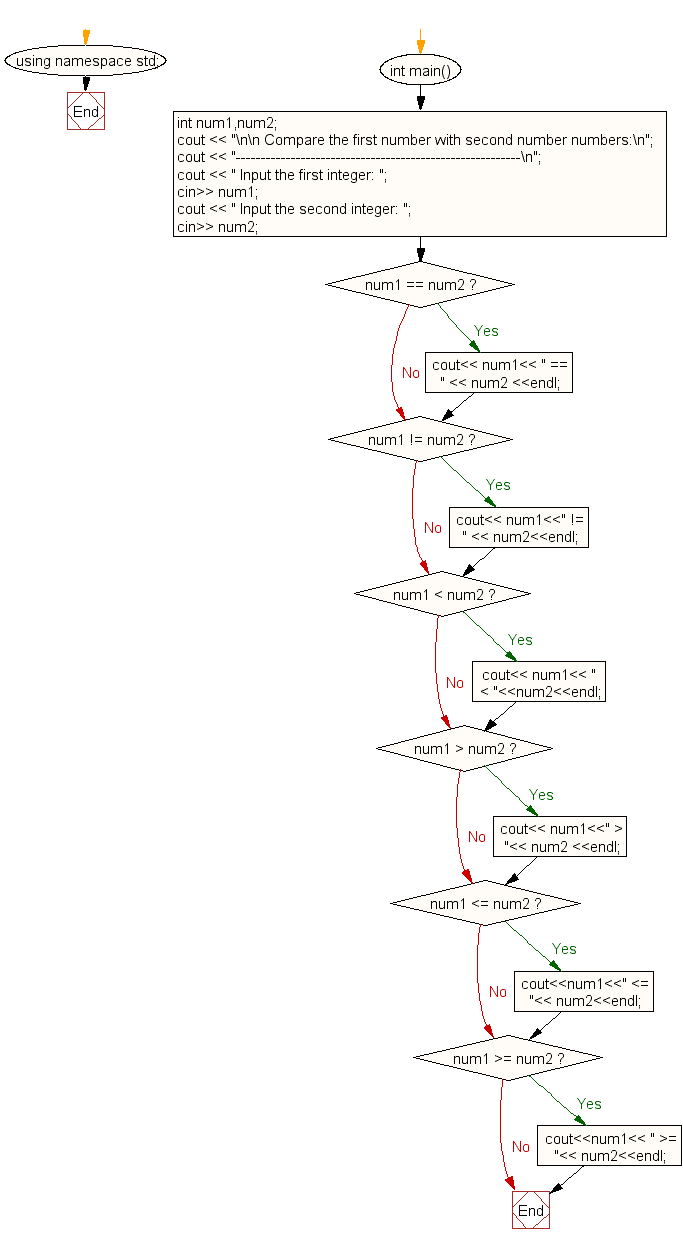﻿ C++ Exercises: Compare two numbers - w3resource# C++ Exercises: Compare two numbers

## C++ For Loop: Exercise-82 with Solution

Write a program in C++ to compare two numbers.

Sample Solution:-

C++ Code :

``````#include <iostream>
using namespace std;

int main()
{
int num1,num2;
cout << "\n\n Compare the first number with second number numbers:\n";
cout << "---------------------------------------------------------\n";
cout << " Input the first integer: ";
cin>> num1;
cout << " Input the second integer: ";
cin>> num2;

if ( num1 == num2 )
cout<< num1<< " == " << num2 <<endl;
if ( num1 != num2 )
cout<< num1<<" != " << num2<<endl;
if ( num1 < num2 )
cout<< num1<< " < "<<num2<<endl;
if ( num1 > num2 )
cout<< num1<<" > "<< num2 <<endl;
if ( num1 <= num2 )
cout<<num1<<" <= "<< num2<<endl;
if ( num1 >= num2 )
cout<<num1<< " >= "<< num2<<endl;
}
``````

Sample Output:

``` Compare the first number with second number numbers:
---------------------------------------------------------
Input the first integer: 25
Input the second integer: 15
25 != 15
25 > 15
25 >= 15
```

Flowchart:C++ Code Editor:

Contribute your code and comments through Disqus.

What is the difficulty level of this exercise?

﻿